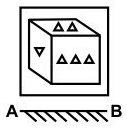Question 24

# If a mirror is placed on the line AB, then which of the answer figure is the right image of the given figure?Solution

A horizontal mirror is placed, so the figure will be reversed, that is, the triangles pointing upwards will face down and vice-versa, and thus the second and third options are not possible.

Also, the triangle on the left will remain at the left hand side in the output, so the last option is also eliminated.

Thus, first is the correct output.

=> Ans - (A)